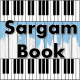# Maps Sargam Notes Maroon 5

Maps Sargam Notes Maroon 5 Available On Sargam Book. Maps Easy Harmonium Notes For Beginners By Maroon 5. Maps Easy Harmonium Notes On Sargam Book By Maroon 5.

Song : Maps
Singer : Maroon 5
Classical Sargam Notes : www.sargambook.com
Guitar / Piano Chords : https://chords.pianodaddy.com

I miss the taste of a sweeter life
Dha(k) Dha(k) Dha(k), Ni Dha(k) Dha(k) Ma(t)
Ma(t) Dha(k) Dha(k)
I miss the conversation
Dha(k) Dha(k) Dha(k)
Ma(t) Ga Ma(t) Dha(k)
I’m searching for a song tonight
Dha(k) Dha(k) Dha(k)
Ni Dha(k) Ma(t) Ga Re(k)
I’m changing all of the stations
Ga Ga Ga, Ma(t) Ga Ga(k) Re(k)

I like to think that we had it all
Dha(k) Dha(k) Dha(k), Ni Dha(k) Dha(k) Ma(t)
Ma(t) Dha(k) Dha(k)
We drew a map to a better place
Dha(k) Dha(k) Dha(k)
Ma(t) Ma(t) Dha(k) Ma(t) Ga
Ma(t) Dha(k) Dha(k)
But on that road I took a fall
Dha(k) Dha(k) Dha(k), Ni Dha(k) Ma(t) Ga
Ma(t) Ga Re(k)
Oh, baby, why did you run away?
Ga Ga Ga, Ma(t) Ma(t) Ga(k)
Ga Ga(k) Ga(k) Re(k)

I was there for you
Ga Ga(k) Re(k), Re(k) Re(k)
Re(k) ni Re(k) Re(k), Re(k)
I was there for you
Ga Ga(k) Re(k), Re(k) Re(k)
Re(k) ni Re(k), Re(k) ni dha(k)

But I wonder, where were you?
When I was at my worst
Down on my knees
And you said you had my back
So I wonder, where were you?
When all the roads you took came back to me

These are demo notes for respective song. You can try it on your instrument. If it works for you and you are comfortable to play along with our notes, you can simply get full notes by paying us. Just click the Buy Now button below and see our packages.#### Titiksha

Titiksha Telang is an Indian Musician & Singer. She is MA in Indian Classical Music & Owner of Sargam Book Website.